Goseeko blog# What is an Instrumentation Amplifier? Definition, working, and advantages

There are three operational amplifiers in the circuit of an Instrumentation Amplifier. The input of the differential amplifier is a non-inverting amplifier. For exact measurement of each input data from transduces this circuit provides high input impedance. The basic circuit for instrumentation amplifiers is shown.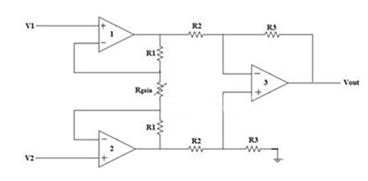The input stage of the inverting amplifier is formed by connecting two non-inverting amplifiers represented as op-amps 1 and 2 in the above figure. The output stage of the instrumentation amplifier is a difference amplifier which is the op-amp 3 in the figure above.

### Working of Instrumentation Amplifier

The output voltage Vout of the difference amplifier is actually the output stage of the instrumentation amplifier. This output voltage Vout is then the difference between the input signals applied at the input terminals.

Let us consider  Vo1 and Vo2 the output voltages of the two op-amps 1 and 2 respectively. Then the value of  voltage Vout of difference amplifier is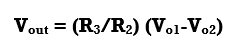The value of voltages Vo1 and Vo2 is in terms of the input voltages and resistances. The input stage of the instrumentation amplifier is also shown below.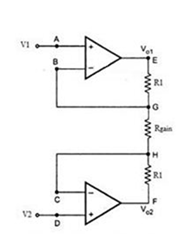Consider the value of input voltage as V1 at node A. From the virtual ground concept, the value of the voltage at node B will be V1 . At node G the potential will also be equal to V1

Consider the value of input voltage as V2 at node D. From the virtual ground concept the value of the voltage at node C will be V2 . At node H the potential will also be equal to V2

The value of current at input stage is zero because we consider the ideal case. Therefore, the value of current remains same in all the resistors R1, Rgain and R1

At node E and F from Ohm’s Law, we can write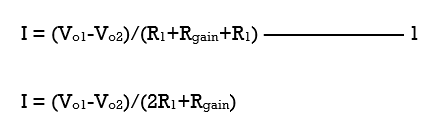As there is no input current at the input stage hence, value of current between node G and H will be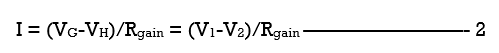Equating both equations 1 and 2,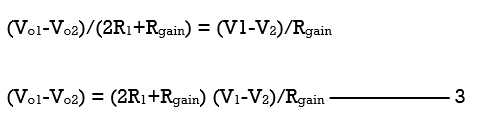The output of the difference amplifier is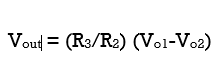Therefore,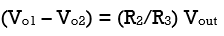In equation 3 we substitute (Vo1 – Vo2) value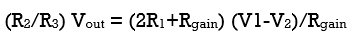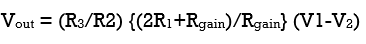The output voltage of an instrumentation amplifier is in the above equation. The value of the overall gain of the amplifier is (R3/R2) {(2R1+Rgain)/Rgain}.